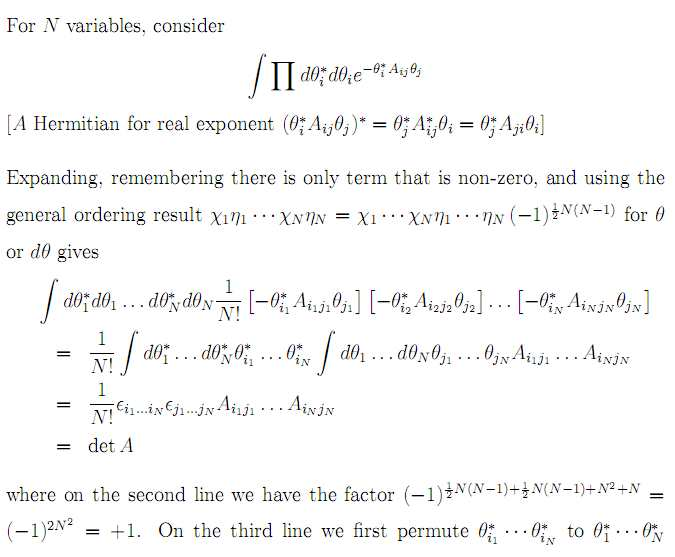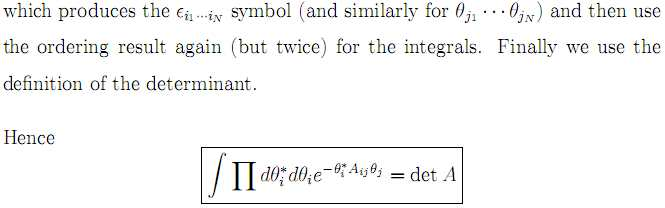# Gaussian Integral Identity with Grassmann Numbers

#### Phileas.Fogg

Hi,
I read the chapter "Anticommuting Numbers" by Peskin & Schröder (page 299) about Grassmann Numbers and now I would like to prove

$$\int d \bar{\theta}_1 d \theta_1 ... d \bar{\theta}_N d \theta_N e^{-\bar{\theta} A \theta} = det A$$

$$\theta_i$$ are complex Grassmann Numbers.

$$\bar{\theta}_i$$ are the complex conjugates of $$\theta_i$$.

In Peskin & Schröder there is no derivation at all, so I tried to find it via google.

In lecture notes I foundBut I don't understand, how the author expanded to get the first line. How he used the general ordering result and permutations to get the rest.

Could anybody explain that or refer to a website, with an explicit example, for maybe N=2 ?

Regards,
Mr. Fogg#### CompuChip

Homework Helper
At first this calculation may be a bit hard to understand, but it exhibits a lot of features which make calculating with Grassmann numbers very straightforward (and tricks you might want to remember)

So first the author is expanding the exponent. As you know,
$$e^x = 1 + x + x^2 / 2 + \cdots + x^n / n! + \cdots$$
In this case,
$$e^{\theta_i^* A_{ij} \theta_j} = 1 + \theta_i A_{ij} \theta_j + (\theta_i^* A_{ij} \theta_j)^2 / 2 + \cdots + (\theta_i^* A_{ij} \theta_j)^n / n! + \cdots$$

Note that inside each pair of brackets, there is a summation going on. If I write out the second term:
$$(\theta_i^* A_{ij} \theta_j)^2 = \left( \sum_{i, j} \theta_i^* A_{ij} \theta_j \right)^2 = \left( \sum_{i_1, j_1} \theta_{i_1}^* A_{i_1 j_1} \theta_{j_1} \right) \left( \sum_{i_2, j_2} \theta_{i_2}^* A_{i_2j_2} \theta_{j_2} \right) = \sum_{i_1, i_2, j_1, j_2} \left[ \left( \theta_{i_1}^* A_{i_1j_1} \theta_{j_1} \right) \left( \theta_{i_2}^* A_{i_2 j_2} \theta_{j_2} \right) \right]$$

Now note, that when we integrate this term, it vanishes. We have N integrals over a theta, and N over a theta*, but the quadratic term which I wrote out only contains 2 of each. So even if the term doesn't vanish beforehand (because $i_1 = i_2$ for example, so there are two identical Grassmann numbers) we can start calculating the integrals and at some point we will integrate over a theta or theta* which is not in the expression.

On the other hand, we only have N theta's and theta*'s. So if we take the (N+m)th power (with m > 0) and expand the power, then we will get sums looking like (for m = 1)
$$\theta_{i_1}^* A_{i_1j_1} \theta_{j_1} \theta_{i_2}^* A_{i_2j_2} \theta_{j_2} \cdots \theta_{i_N}^* A_{i_Nj_N} \theta_{j_N} \theta_{i_{N+1}}^* A_{i_{N+1}j_{N+1}} \theta_{j_{N+1}}$$
in which there must necessarily be two equal theta's and two equal theta^*'s, so it vanishes.

On the second line, the author is just permuting stuff (pulling each theta through a theta or theta* gives a minus sign, a theta* through a theta or theta* gives a minus sign, and a theta or theta* through an A gives a plus sign - assuming that the matrix is real-valued and not Grassmann-valued) so from line 1 to line 2 you get at most a sign change (which is explained to be + in the text below).

#### Phileas.Fogg

Thank You very much for the explanation.

On the other hand, we only have N theta's and theta*'s. So if we take the (N+m)th power (with m > 0) and expand the power, then we will get sums looking like (for m = 1)
$$\theta_{i_1}^* A_{i_1j_1} \theta_{j_1} \theta_{i_2}^* A_{i_2j_2} \theta_{j_2} \cdots \theta_{i_N}^* A_{i_Nj_N} \theta_{j_N} \theta_{i_{N+1}}^* A_{i_{N+1}j_{N+1}} \theta_{j_{N+1}}$$
in which there must necessarily be two equal theta's and two equal theta^*'s, so it vanishes.
To my better understanding, I try to do the proof for N=2

$$\int d \theta_1^* d \theta_1 d \theta_2^* d \theta_2 (-(1 + \theta_i^* A_{ij} \theta_j + (\theta_i^* A_{ij} \theta_j)^2 / 2 + \cdots + (\theta_i^* A_{ij} \theta_j)^n / n! + \cdots ))$$

Since the quadratic term and all higher powers vanish, I get

$$\int d \theta_1^* d \theta_1 d \theta_2^* d \theta_2 (-(1 + \sum_{i, j} \theta_i^* A_{ij} \theta_j))$$

The Integral over 1 is zero.

$$\int d \theta_1^* d \theta_1 d \theta_2^* d \theta_2 (- \sum_{i, j} \theta_i^* A_{ij} \theta_j) = \int d \theta_1^* d \theta_1 d \theta_2^* d \theta_2 (- \theta_1^* A_{11} \theta_1 - \theta_2^* A_{21} \theta_2 - \theta_1^* A_{12} \theta_2 - \theta_2^* A_{22} \theta_2)$$

Is that correct?

Regards,
Mr. Fogg

#### CompuChip

Homework Helper
The quadratic term does not vanish. You can explicitly work out the sum for n = 1:
$$\theta_i^* A_{ij} \theta_j = \theta_1^* A_{11} \theta_1 + \theta_1^* A_{12} \theta 2 + \theta_2^* A_{21} \theta_1 + \theta_2^* A_{22} \theta_2$$

Now square that, what do you get?

Also, what happens when you explicitly do your integration
$$\int d \theta_1^* d \theta_1 d \theta_2^* d \theta_2 (- \theta_1^* A_{11} \theta_1 - \theta_2^* A_{21} \theta_2 - \theta_1^* A_{12} \theta_2 - \theta_2^* A_{22} \theta_2)$$

Last edited:

#### Phileas.Fogg

The quadratic term does not vanish. You can explicitly work out the sum for n = 1:
$$\theta_i^* A_{ij} \theta_j = \theta_1^* A_{11} \theta_1 + \theta_1^* A_{12} \theta 2 + \theta_2^* A_{21} \theta_1 + \theta_2^* A_{22} \theta_2$$

Now square that, what do you get?
Using $$(\theta_i)^2 = (\theta_i^*)^2 = 0$$

I get
$$(\theta_1^* A_{11} \theta_1) (\theta_2^* A_{22} \theta_2) + (\theta_1^* A_{12} \theta_2)(\theta_2^* A_{21} \theta_1) + (\theta_2^* A_{21} \theta_1)(\theta_1^* A_{12} \theta_2) + (\theta_2^* A_{22} \theta_2)(\theta_1^* A_{11} \theta_1)$$

But isn't this the case N = 2 , because i and j run till 2 ?

Also, what happens when you explicitly do your integration
$$\int d \theta_1^* d \theta_1 d \theta_2^* d \theta_2 (- \theta_1^* A_{11} \theta_1 - \theta_2^* A_{21} \theta_2 - \theta_1^* A_{12} \theta_2 - \theta_2^* A_{22} \theta_2)$$
I will do this integration tomorrow morning, because I have to leave now.

Thanks for your help!

Mr. Fogg

#### CompuChip

Homework Helper
To my better understanding, I try to do the proof for N=2
[...]
[...]
But isn't this the case N = 2 , because i and j run till 2 ?
Precisely.

Now observe how you have to be careful with sums. A simple expression like $\theta_1 \theta_2^*$ will vanish when squared, but sums of such terms do not necessarily (although stuff will cancel out).

[...]
I will do this integration tomorrow morning, because I have to leave now.
It's really easy, you'll see.
Don't bring out the cigar yet, though... the square term is what you are interested in (see the general proof).

[...]
Thanks for your help!
Welcome.

#### Phileas.Fogg

The integration
$$\int d \theta_1^* d \theta_1 d \theta_2^* d \theta_2 (- \theta_1^* A_{11} \theta_1 - \theta_2^* A_{21} \theta_2 - \theta_1^* A_{12} \theta_2 - \theta_2^* A_{22} \theta_2)$$
gives
$$- \int d \theta_1^* d \theta_1 (\theta_1^* A_{11} \theta_1) - \int d \theta_2^* d \theta_2 (\theta_2^* A_{21} \theta_2) - \int d \theta_1^* d \theta_2 (\theta_1^* A_{12} \theta_2) - \int d \theta_2^* d \theta_2 (\theta_2^* A_{22} \theta_2)$$

$$A_{11} + A_{21} + A_{12} + A_{22}$$

There must be a mistake, because I don't see, how this helps me.
-----------------------------------------------------
If I work out the quadratic term explicitly, square it and do the integration (like above), I get

$$A_{11} A_{22} - A_{12} A_{21} + A_{21} A_{12} + A_{22} A_{11}$$

This looks a little more like a determinant, but there are some terms too much in this expression. Where is the mistake here?

Regards,
Mr. Fogg

#### samwise

The integration
$$\int d \theta_1^* d \theta_1 d \theta_2^* d \theta_2 (- \theta_1^* A_{11} \theta_1 - \theta_2^* A_{21} \theta_2 - \theta_1^* A_{12} \theta_2 - \theta_2^* A_{22} \theta_2)$$
gives
$$- \int d \theta_1^* d \theta_1 (\theta_1^* A_{11} \theta_1) - \int d \theta_2^* d \theta_2 (\theta_2^* A_{21} \theta_2) - \int d \theta_1^* d \theta_2 (\theta_1^* A_{12} \theta_2) - \int d \theta_2^* d \theta_2 (\theta_2^* A_{22} \theta_2)$$

$$A_{11} + A_{21} + A_{12} + A_{22}$$
Remember that you are also integrating over the variables not in the expression. For example, the first term is actually
$$\int d \theta_1^* d \theta_1 d \theta_2^* d \theta_2 (\theta_1^* A_{11} \theta_1) = - A_{11} \int d \theta_1^* \theta_1^* d \theta_1 \theta_1 \int d \theta_2^* d \theta_2 = 0$$
since
$$\int d \theta_2 = 0 = \int d \theta_2^*$$
so you get zero for all those terms, and the only contributions to the integral come from the quadratic terms (where you can get terms that include all 4 variables and do not vanish). Higher than quadratic terms, in this case, contain squares and therefore vanish.

Also, I think your term
$$\theta_2^* A_{21} \theta_2$$ should read $$\theta_2^* A_{21} \theta_1$$

For the quadratic term, you should get
$$2(A_{11} A_{22} - A_{12} A_{21}) = 2 detA$$ where the $$2$$ cancels the factor $$\frac{1}{2!}$$ brought down with the expansion of the exponential. The sign difference you have on your $$A_{21} A_{12}$$ could be due to your summation where you have $$\theta_2^* A_{21} \theta_2$$ instead of $$\theta_2^* A_{21} \theta_1$$.

Regards,
samwise.

Last edited:

#### CompuChip

Homework Helper
Note that in doing the integration, you need to re-order the terms. I don't readily see whether you took that into account, but for example:
$$\int d \theta_1^* d \theta_1 d \theta_2^* d \theta_2 \theta_1^* A_{12} \theta_2 \theta_2^* A_{21} \theta_1 = A_{12} A_{21} \int d \theta_1^* d \theta_1 d \theta_2^* d \theta_2 \theta_1^* \theta_2 \theta_2^* \theta_1 = (-1)^{2+1} A_{12} A_{21} \int d \theta_1^* d \theta_1 d \theta_2^* d \theta_2 \theta_1^* \theta_1\theta_2^*\theta_2 = - A_{12} A_{21}$$
where the factors of (-1) arise from ordering the theta's.
Which way you should order them depends on how Grassmann numbers are introduced, you can require that the theta's be in the same order as the integrals, so
$$\int d\theta_1 d\theta_2 \cdots d\theta_n \theta_1 \theta_2 \cdots \theta_n = 1$$
or that each theta has to be brought to its corresponding integration, so
$$\int d\theta_1 d\theta_2 \cdots d\theta_n \theta_n \theta_{n-1} \cdots \theta_2 \theta_1 = 1$$
(first do the inner integration, etc).

This will produce some minus signs. Then the result can be simplified, because the A's are just numbers, so
$$A_{11} A_{22} + A_{22} A_{11} = 2 A_{11} A_{22}$$.

#### Phileas.Fogg

Hello,
thanks for Your help!

Also, I think your term
$$\theta_2^* A_{21} \theta_2$$ should read $$\theta_2^* A_{21} \theta_1$$

For the quadratic term, you should get
$$2(A_{11} A_{22} - A_{12} A_{21}) = 2 detA$$ where the $$2$$ cancels the factor $$\frac{1}{2!}$$ brought down with the expansion of the exponential. The sign difference you have on your $$A_{21} A_{12}$$ could be due to your summation where you have $$\theta_2^* A_{21} \theta_2$$ instead of $$\theta_2^* A_{21} \theta_1$$.
In the quadratic term, I didn't make the same mistake with the indices.

To do the integral of the quadratic term, I used

$$\int d \theta^* d \theta \theta^* \theta = -1$$

Then I get

$$- \frac{1}{2} (A_{11}A_{22} - A_{12}A_{21} - A_{21}A_{12} + A_{22}A_{11})$$

The minus sign is left from the expansion.

Is $$A_{12}A_{21} = A_{21}A_{12} \; \text{and} \; A_{11}A_{22} = A_{22}A_{11}?$$

If that was true and I didn't have the minus sign, then it would be Samwise's solution.

What is right now?

Regards,
Mr. Fogg

#### Avodyne

Is $$A_{12}A_{21} = A_{21}A_{12} \; \text{and} \; A_{11}A_{22} = A_{22}A_{11}?$$
Yes, the elements of the A matrix are assumed to be commuting numbers.
If that was true and I didn't have the minus sign, then it would be Samwise's solution.
The overall sign doesn't matter; it depends on the convention for ordering the differentials.

### The Physics Forums Way

We Value Quality
• Topics based on mainstream science
• Proper English grammar and spelling
We Value Civility
• Positive and compassionate attitudes
• Patience while debating
We Value Productivity
• Disciplined to remain on-topic
• Recognition of own weaknesses
• Solo and co-op problem solving